HomeTutorialsDeep Learning

# Demystifying Generative Adversarial Nets (GANs)

Learn what Generative Adversarial Networks are without going into the details of the math and code a simple GAN that can create digits!
May 2018  · 9 min read

In this tutorial, you will learn what Generative Adversarial Networks (GANs) are without going into the details of the math. After, you will learn how to code a simple GAN which can create digits!

## Analogy

The easiest way to understand what GANs are is through a simple analogy:

Suppose there is a shop which buys certain kinds of wine from customers which they will later resell.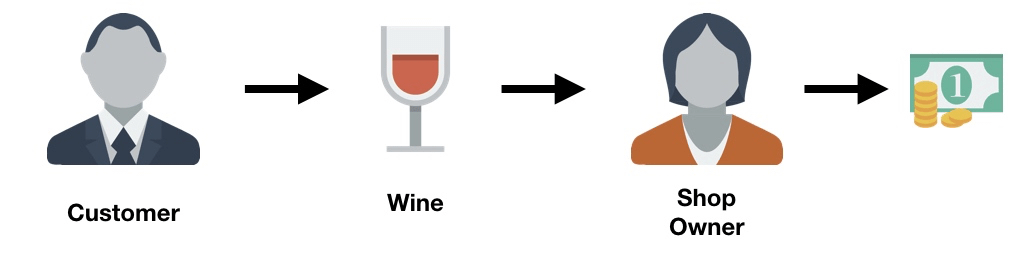However, there are nefarious customers who sell fake wine in order to get money. In this case, the shop owner has to be able to distinguish between the fake and authentic wines.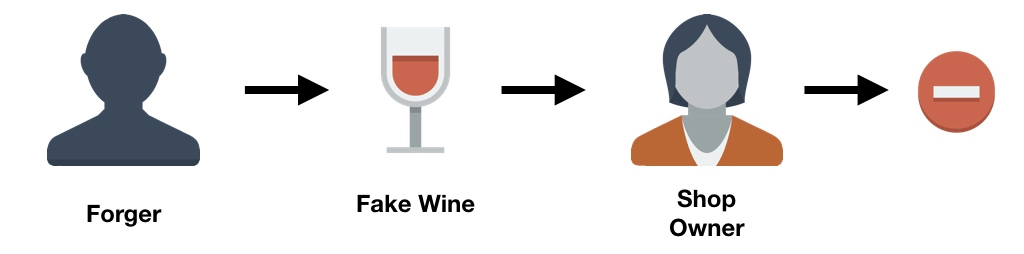You can imagine that initially, the forger might make a lot of mistakes when trying to sell the fake wine and it will be easy for the shop owner to identify that the wine is not authentic. Because of these failures, the forger will keep on trying different techniques to simulate the authentic wines and some will eventually be successful. Now that the forger knows that certain techniques got past the shop owner's checks, he can start to further improve the fake wines based on those techniques.

At the same time, the shop owner would probably get some feedback from other shop owners or wine experts that some of the wines that she has are not original. This means that the shop owner would have to improve how she determines whether a wine is fake or authentic. The goal of the forger is to create wines that are indistinguishable from the authentic ones, and the goal of the shop owner is to accurately tell if a wine is real or not.

This back-and-forth competition is the main idea behind GANs.

## Components of a Generative Adversarial Network

Using the example above, we can come up with the architecture of a GAN.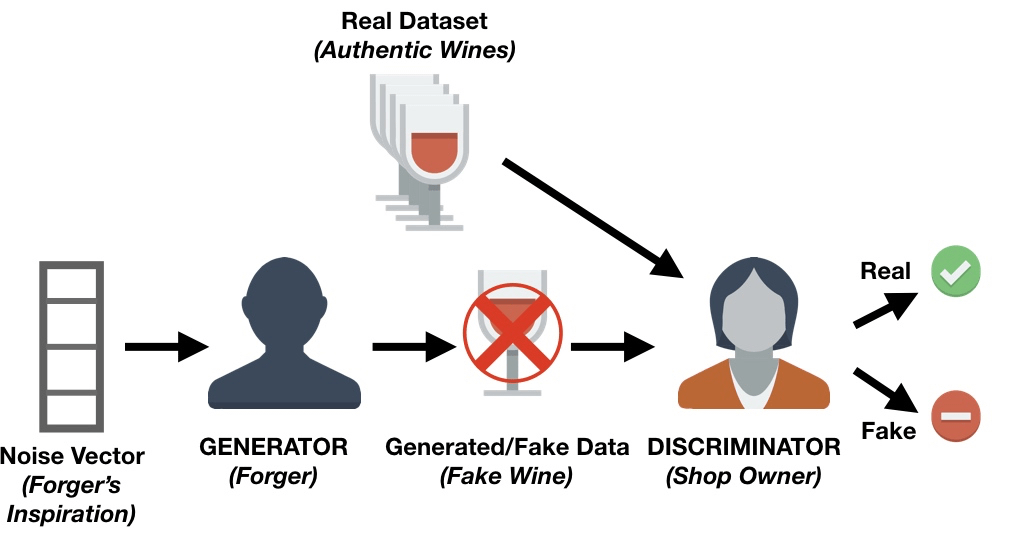There are two major components within GANs: the generator and the discriminator. The shop owner in the example is known as a discriminator network and is usually a convolutional neural network (since GANs are mainly used for image tasks) which assigns a probability that the image is real.

The forger is known as the generative network, and is also typically a convolutional neural network (with deconvolution layers). This network takes some noise vector and outputs an image. When training the generative network, it learns which areas of the image to improve/change so that the discriminator would have a harder time differentiating its generated images from the real ones.

The generative network keeps producing images that are closer in appearance to the real images while the discriminative network is trying to determine the differences between real and fake images. The ultimate goal is to have a generative network that can produce images which are indistinguishable from the real ones.

## A Simple Generative Adversarial Network with Keras

Now that you understand what GANs are and the main components of them, we can now begin to code a very simple one. You will use `Keras` and if you are not familiar with this Python library you should read this tutorial before you continue. This tutorial is based on the GAN developed here.

The first thing you would need to do is install the following packages via `pip`

• `keras`
• `matplotlib`
• `tensorflow`
• `tqdm`

You will use `matplotlib` for plotting, `tensorflow` as the Keras backend library and `tqdm` to show a fancy progress bar for each epoch (iteration).

The next step is to create a Python script. In this script, you will first need to import all the modules and functions you will use. An explanation of each will be given as they are used.

``````import os
import numpy as np
import matplotlib.pyplot as plt
from tqdm import tqdm

from keras.layers import Input
from keras.models import Model, Sequential
from keras.layers.core import Dense, Dropout
from keras.datasets import mnist
from keras import initializers
``````

You now want to set some variables:

``````# Let Keras know that we are using tensorflow as our backend engine
os.environ["KERAS_BACKEND"] = "tensorflow"

# To make sure that we can reproduce the experiment and get the same results
np.random.seed(10)

# The dimension of our random noise vector.
random_dim = 100
``````

Before you can start building the discriminator and generator, you should first gather and pre-process the data. You will use the popular MNIST dataset which has a set of images of single digits ranging from 0 to 9.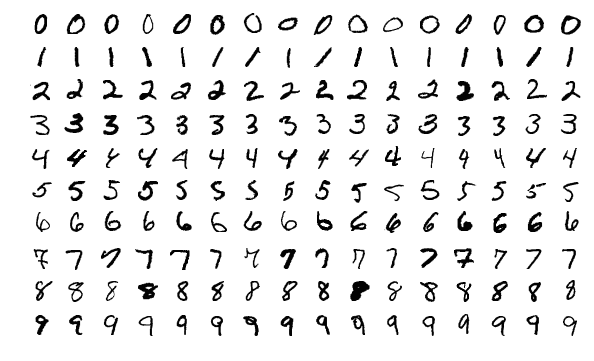``````def load_minst_data():
(x_train, y_train), (x_test, y_test) = mnist.load_data()
# normalize our inputs to be in the range[-1, 1]
x_train = (x_train.astype(np.float32) - 127.5)/127.5
# convert x_train with a shape of (60000, 28, 28) to (60000, 784) so we have
# 784 columns per row
x_train = x_train.reshape(60000, 784)
return (x_train, y_train, x_test, y_test)
``````

Note that the `mnist.load_data()` is part of Keras and allows you to easily import the MNIST dataset into your workspace.

Now, you can create your generator and discriminator networks. You will use the Adam optimizer for both networks. For both the generator and discriminator, you will create a neural network with three hidden layers with the activation function being the Leaky Relu. You should also add dropout layers for the discriminator to improve its robustness on unseen images.

``````# You will use the Adam optimizer
def get_optimizer():

def get_generator(optimizer):
generator = Sequential()

generator.compile(loss='binary_crossentropy', optimizer=optimizer)
return generator

def get_discriminator(optimizer):
discriminator = Sequential()

discriminator.compile(loss='binary_crossentropy', optimizer=optimizer)
return discriminator
``````

It is finally time to bring the generator and discriminator together!

``````def get_gan_network(discriminator, random_dim, generator, optimizer):
# We initially set trainable to False since we only want to train either the
# generator or discriminator at a time
discriminator.trainable = False
# gan input (noise) will be 100-dimensional vectors
gan_input = Input(shape=(random_dim,))
# the output of the generator (an image)
x = generator(gan_input)
# get the output of the discriminator (probability if the image is real or not)
gan_output = discriminator(x)
gan = Model(inputs=gan_input, outputs=gan_output)
gan.compile(loss='binary_crossentropy', optimizer=optimizer)
return gan
``````

For completeness, you can create a function which will save your generated images every 20 epochs. Since this is not at the core of this lesson, you do not need to fully understand the function.

``````# Create a wall of generated MNIST images
def plot_generated_images(epoch, generator, examples=100, dim=(10, 10), figsize=(10, 10)):
noise = np.random.normal(0, 1, size=[examples, random_dim])
generated_images = generator.predict(noise)
generated_images = generated_images.reshape(examples, 28, 28)

plt.figure(figsize=figsize)
for i in range(generated_images.shape):
plt.subplot(dim, dim, i+1)
plt.imshow(generated_images[i], interpolation='nearest', cmap='gray_r')
plt.axis('off')
plt.tight_layout()
plt.savefig('gan_generated_image_epoch_%d.png' % epoch)
``````

You have now coded the majority of your network. All that remains is to train this network and take a look at the images that you created.

``````def train(epochs=1, batch_size=128):
# Get the training and testing data
x_train, y_train, x_test, y_test = load_minst_data()
# Split the training data into batches of size 128
batch_count = x_train.shape / batch_size

# Build our GAN netowrk
gan = get_gan_network(discriminator, random_dim, generator, adam)

for e in xrange(1, epochs+1):
print '-'*15, 'Epoch %d' % e, '-'*15
for _ in tqdm(xrange(batch_count)):
# Get a random set of input noise and images
noise = np.random.normal(0, 1, size=[batch_size, random_dim])
image_batch = x_train[np.random.randint(0, x_train.shape, size=batch_size)]

# Generate fake MNIST images
generated_images = generator.predict(noise)
X = np.concatenate([image_batch, generated_images])

# Labels for generated and real data
y_dis = np.zeros(2*batch_size)
# One-sided label smoothing
y_dis[:batch_size] = 0.9

# Train discriminator
discriminator.trainable = True
discriminator.train_on_batch(X, y_dis)

# Train generator
noise = np.random.normal(0, 1, size=[batch_size, random_dim])
y_gen = np.ones(batch_size)
discriminator.trainable = False
gan.train_on_batch(noise, y_gen)

if e == 1 or e % 20 == 0:
plot_generated_images(e, generator)

if __name__ == '__main__':
train(400, 128)
``````

After training for 400 epochs, you can view the images that were generated. Looking at the images produced after the first epoch, you can see that it does not have any real structure, looking at the image after 40 epochs, the digits start to take shape and lastly, the images produced after 400 epochs show clear digits even though a couple are still unrecognizable.

Results after 1 epoch Results after 40 epochs Results after 400 epochs
!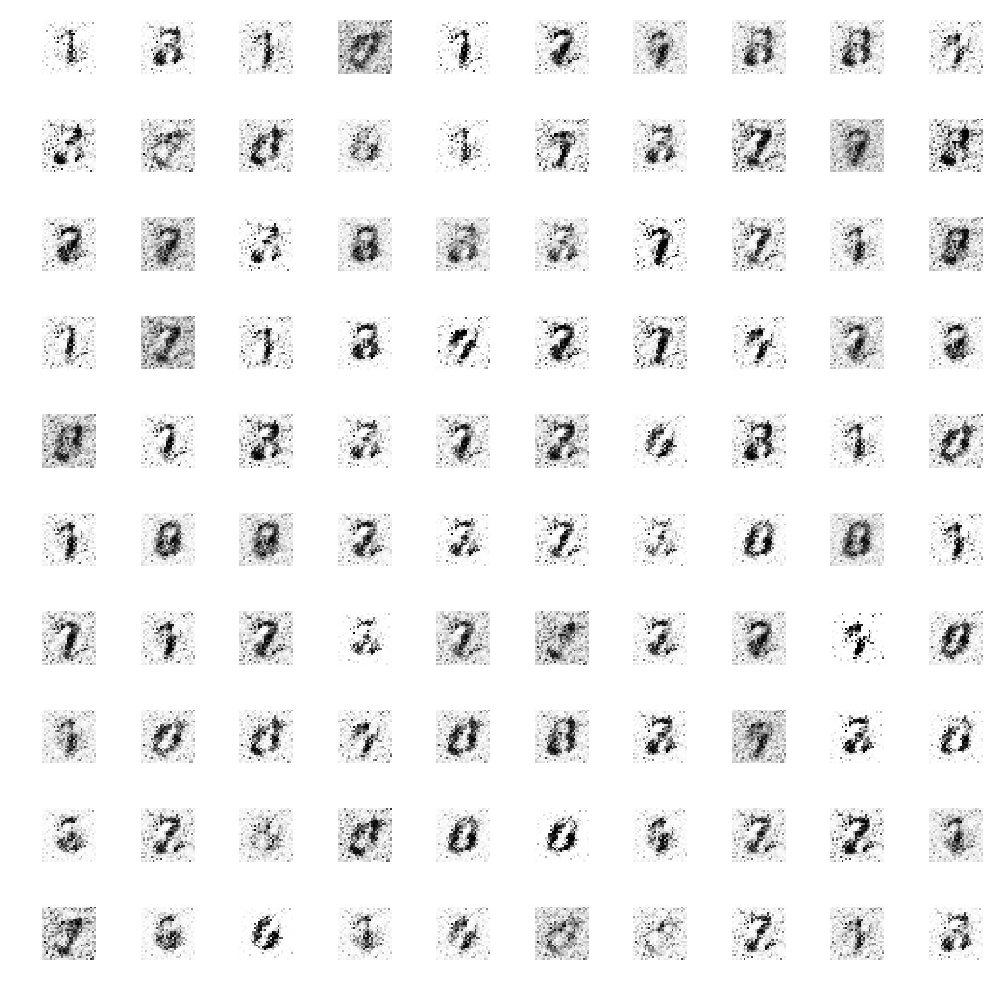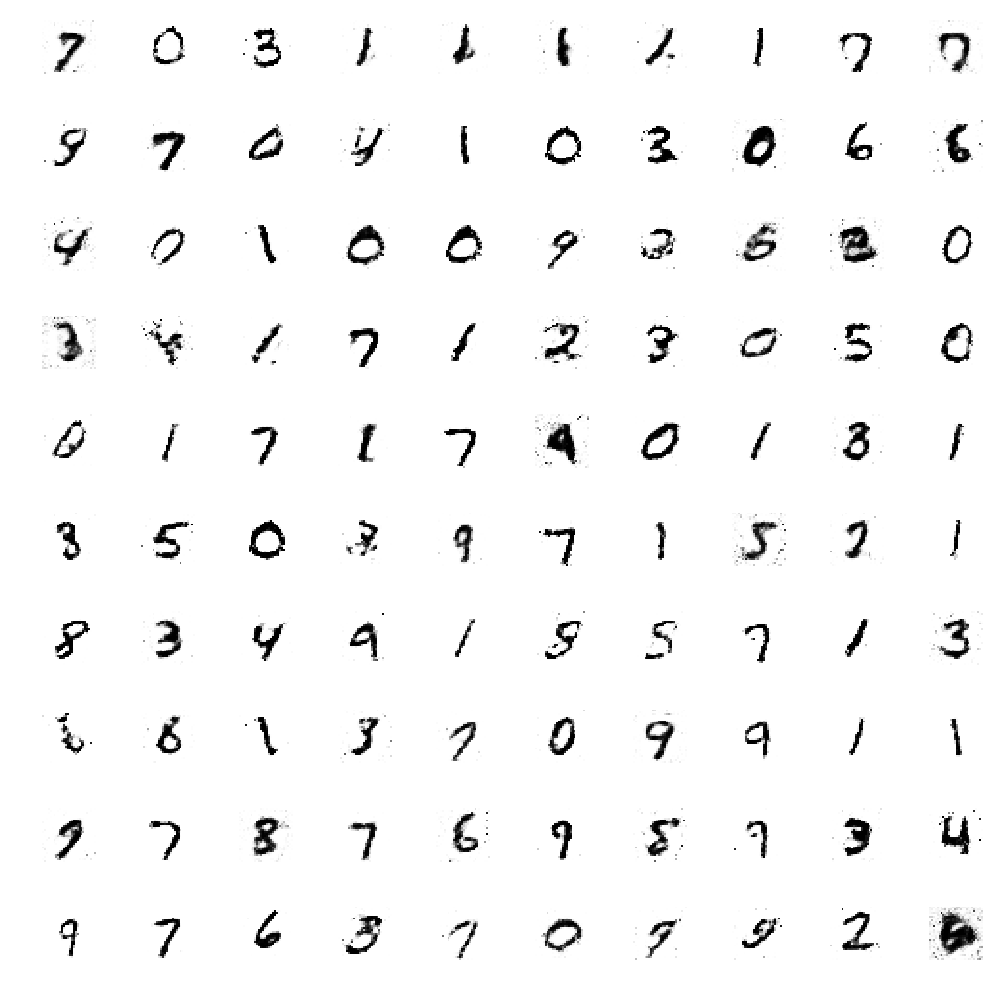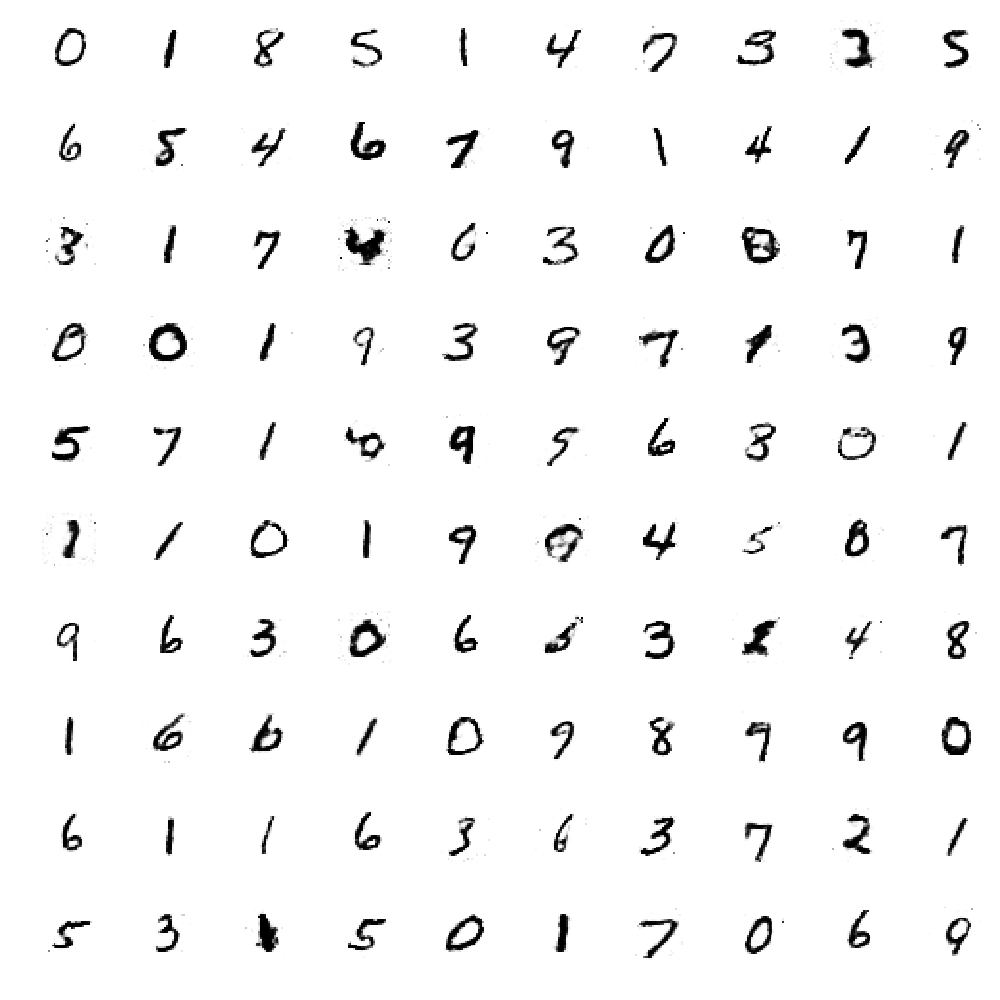This code takes approximately 2 minutes per epoch on a CPU and that is the main reason this code was selected. You can experiment by using more epochs and by adding more (and different) layers to both the generator and discriminator. However, when using more complex and deep architectures the runtime will also increase if you are using only a CPU. However, do not let that stop you from experimenting!

## Conclusion

Congrats, you've made it to the end of this tutorial, in which you learned the basics of Generative Adversarial Networks (GANs) in an intuitive way! Also, you implemented your first model with the help of the Keras library. If you want to know more about deep learning with Python, consider taking DataCamp's Deep Learning in Python course.

### .css-1531qan{-webkit-text-decoration:none;text-decoration:none;color:inherit;}Introduction to Deep Learning in Python

BeginnerSkill Level
4 hr
240.3K
Learn the fundamentals of neural networks and how to build deep learning models using Keras 2.0 in Python.
See Details
Start Course

### Image Processing with Keras in Python

BeginnerSkill Level
4 hr
33.4K
Learn to conduct image analysis using Keras with Python by constructing, training, and evaluating convolutional neural networks.

### Advanced Deep Learning with Keras

BeginnerSkill Level
4 hr
30.1K
Build multiple-input and multiple-output deep learning models using Keras.
See More
Related### Announcing the "Become an AI Developer" Code-Along Series

Get started with Generative AI in this brand new code-along series. Free for a limited time.DataCamp Team

4 min### ChatGPT & Generative AI: The Year in Review – Top 17 Moments

Explore the pivotal year for ChatGPT and generative AI with our comprehensive review of 2023's top 17 AI milestones.### Data & AI for Good, with Marga Hoek, Founder & CEO, Business for Good

Marga and Adel explore the fourth industrial revolution, how data and AI enable real-time information sharing, use cases of tech for good initiatives, how collaboration can bridge the gap in investment for sustainable business ventures and a lot more.

### How to Make Custom ChatGPT Models: 5 Easy Steps to Personalized GPTs

Check out these five simple steps to unlock the full potential of ChatGPT with your own custom GPTs.

### Fine-tuning Stable Diffusion XL with DreamBooth and LoRA

Learn how to successfully fine-tune Stable Diffusion XL on personal photos using Hugging Face AutoTrain Advance, DreamBooth, and LoRA for customized, high-quality image generation.

### What is A Confusion Matrix in Machine Learning? The Model Evaluation Tool Explained

A beginner's tutorial to learning about the Confusion Matrix in machine learning.Nisha Arya Ahmed

12 min

See MoreSee More